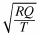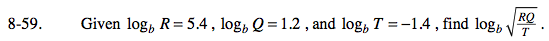### Home > PC > Chapter 8 > Lesson 8.1.4 > Problem8-59

8-59.

Given logb R = 5.4, logb Q = 1.2, and logb T = −1.4, find logb. Homework Help ✎Break this log up into three logs. Then substitute the given values.

$\text{log}_{b}\sqrt{\frac{RQ}{T}}=\text{log}_{b}\left(\frac{RQ}{T}\right)^{\left(\frac{1}{2}\right)}$